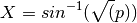# biom_calc module¶

This module provides methods for calculating various metrics with regards to each OTU in an input OTU abundance table.

## arcsine_sqrt_transform¶

Takes the proportion data from relative_abundance() and applies the variance stabilizing arcsine square root transformation:usage: phylotoast.biom_calc.arcsine_sqrt_transform(rel_abd)

rel_abd:

Refers to a dictionary keyed on SampleIDs, and the values are dictionaries keyed on OTUID’s and their values represent the relative abundance of that OTUID in that SampleID. rel_abd is the output of relative_abundance() function.

return:

Returns a dictionary keyed on SampleIDs, and the values are dictionaries keyed on OTUID’s and their values represent the transformed relative abundance of that OTUID in that SampleID.

## mean_otu_pct_abundance¶

Calculate the mean OTU abundance percentage.

usage: phylotoast.biom_calc.mean_otu_pct_abundance(rel_abd, otuIDs)

rel_abd:

Refers to a dictionary keyed on SampleIDs, and the values are dictionaries keyed on OTUID’s and their values represent the relative abundance of that OTUID in that SampleID. rel_abd is the output of relative_abundance() function.

otuIDs:

A list of OTUID’s for which the percentage abundance needs to be measured.

return:

A dictionary of OTUID and their percent relative abundance as key/value pair.

## MRA¶

Calculate the mean relative abundance.

usage: phylotoast.biom_calc.MRA(biomf)

biomf:

A BIOM file.

return:

A dictionary keyed on OTUID’s and their mean relative abundance for a given number of sampleIDs.

## raw_abundance¶

Calculate the total number of sequences in each OTU or SampleID.

usage: phylotoast.biom_calc.raw_abundance(biomf, sampleIDs=None, sample_abd=True)

biomf:

A BIOM file.

sampleIDs:

A list of column id’s from BIOM format OTU table. By default, the list has been set to None.

sample_abd:

A boolean operator to provide output for OTUID’s or SampleID’s. By default, the output will be provided for SampleID’s.

return:

Returns a dictionary keyed on either OTUID’s or SampleIDs and their respective abundance as values.

## relative_abundance¶

Calculate the relative abundance of each OTUID in a Sample.

usage: phylotoast.biom_calc.relative_abundance(biomf)

biomf:

A BIOM format.

return:

Returns a dictionary keyed on SampleIDs, and the values are dictionaries keyed on OTUID’s and their values represent the relative abundance of that OTUID in that SampleID.

## transform_raw_abundance¶

Function to transform the total abundance calculation for each sample ID to another format based on user given transformation function.

usage: phylotoast.biom_calc.transform_raw_abundance(biomf, fn=math.log10, sampleIDs=None, sample_abd=True)

biomf:

A BIOM file.

fn:

Mathematical function which is used to transform smax to another format. By default, the function has been given as base 10 logarithm.

sampleIDs:

A list of column id’s from BIOM format OTU table. By default, the list has been set to None.

sample_abd:

A boolean operator to provide output for OTUID’s or SampleID’s. By default, the output will be provided for SampleID’s.

return:

Returns a dictionary similar to output of raw_abundance function but with the abundance values modified by the mathematical operation. By default, the operation performed on the abundances is base 10 logarithm.